## Calculate the mass of earth earth assum assuming that it i a 6.67x106m. Sphere with radius​

Question

Calculate the mass of earth
earth assum
assuming
that it i a
6.67x106m.

in progress 0
2 months 2021-07-27T17:24:10+00:00 1 Answers 1 views 0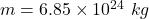Explanation:

The radius of Earth is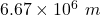The density of an object is given by :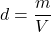The density of Erth is, d = 5515 kg/m³

Where

m is the mass of the Earth

So,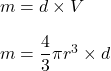Put all the values,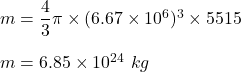So, the mass of the Earth is equal to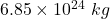.